Questions from the forum
 Q: I want a camera that can zoom in and out, rotates around the player but always faces it. The left and right arrow key should do that; the up and down arrow should move above and below the player. A: Here's an example.   // use WSAD to walk, keys left / right to rotate the camera around the player // use the mouse wheel to zoom in / out and the up / down keys to set the height of the camera action player_cam() // player / camera code {        VECTOR temp;        VECTOR temp2;        var movement_speed = 5; // movement speed        var anim_percentage;        var cam_angle = 90; // set the initial camera angle here        var cam_dist = 250; // set the default zoom in factor here        var cam_height = 150; // set the default camera height here        player = my; // I'm the player        while (1)          {                  if((!key_w) && (!key_s)) // the player isn't moving at all?                    {                        ent_animate(my, "stand", anim_percentage, ANM_CYCLE); // and play the "stand" animation                }                    else // the player is moving?             {                        ent_animate(my, "walk", anim_percentage, ANM_CYCLE); // and play the "walk" animation                    }                // zoom in / out using the mouse wheel                cam_dist += 0.2 * mickey.z * time_step;                // limit the distance between the camera and the player to 20...500                cam_dist = clamp(cam_dist, 20, 500);                // set the height of the camera using the up / down cursor keys                cam_height += 3 * (key_cuu - key_cud) * time_step;                // limit the height of the camera to 50...300                cam_height = clamp(cam_height, 50, 300);                // rotate the player using the A / D keys                my.pan += 4 * (key_a - key_d) * time_step;             anim_percentage += 5 * time_step; // 5 = animation speed                    camera.x = player.x - cam_dist * cos(cam_angle);                camera.y = player.y - cam_dist * sin(cam_angle);             cam_angle += 5 * (key_cur - key_cul) * time_step; // 5 gives the camera rotation speed                camera.z = player.z + cam_height;             vec_set(temp2.x, my.x);             vec_sub(temp2.x, camera.x);             vec_to_angle(camera.pan, temp2); // rotate the camera towards the player at all times             temp.x = movement_speed * (key_w - key_s) * time_step;             temp.y = 0;             temp.z = 0;             c_move (my, temp.x, nullvector, IGNORE_PASSABLE | GLIDE);             wait (1);        } }     Q: How can I use baked textures? A: A baked texture is a bitmap that already contains the effect of the light on its surface. You can use expensive solutions such as Maya / Mental Ray to bake your textures, or even GameStudio to do it. Here's an example:   1) Create a block in Wed and then put your favorite texture on it.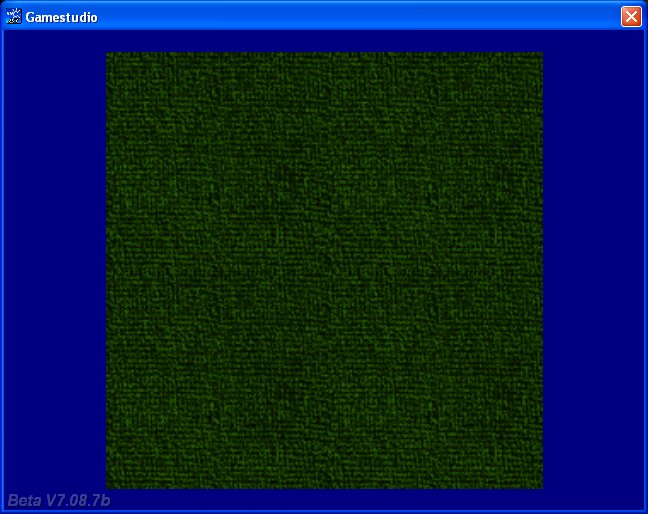2) Add one or more lights close to the surface of the block, and then set their range, color, etc.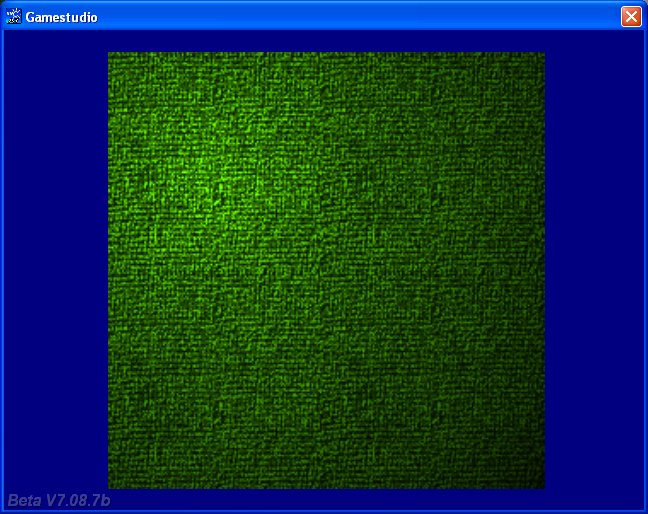3) Capture a screenshot, and then select only the texture from the image.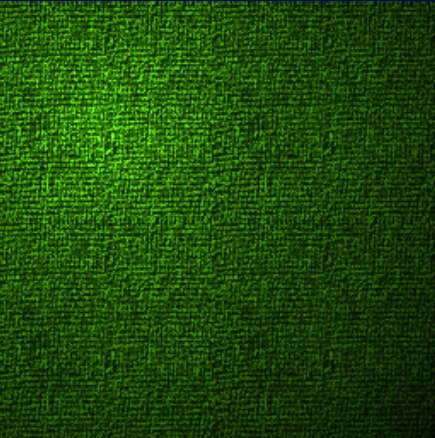4) Add the texture to your wad and use it in your projects; the game will use less video memory if you set the Wed blocks to "Flat".     Q: I would need some code which allows a player to ride upon a moving train on a path, but while the player is on the train he can move around also. A: Use this example as a base for your code.   var entity_speed = 3; var dist_to_node; var current_node = 1; var angle_difference = 0;   VECTOR temp_angle; VECTOR pos_node; // stores the position of the node   ENTITY* train;   function move_target() {        while(1)        {                entity_speed = minv(5, entity_speed + 0.5 * time_step);                    c_move(my, vector(entity_speed * time_step, 0, 0), nullvector, IGNORE_PASSABLE | GLIDE);             vec_to_angle (my.pan, vec_diff (temp_angle, pos_node, my.x));           wait(1);        } }   action move_on_path() // attach this action to your model {        train = my;               set(my, POLYGON);        move_target();        path_scan(me, my.x, my.pan, vector(360, 180, 1000));        path_getnode (my, 1, pos_node, NULL);        vec_to_angle (my.pan, vec_diff (temp_angle, pos_node, my.x)); // rotate towards the node        while(1)        {                dist_to_node = vec_dist(my.x, pos_node);                if(dist_to_node < 50) // close to the node?                {                        current_node = path_nextnode(my, current_node, 1);                        if (!current_node) {current_node = 1;} // reached the end of the path? Then start over!                        path_getnode (my, current_node, pos_node, NULL);                }                wait(1);        } }   action player_over_train() {        while (!train) {wait (1);}        var player_offset_x = 0;        var player_offset_y = 0;        var anim_percentage;        set(my, PASSABLE);        while (1)        {                player_offset_x += 5 * (key_w - key_s) * time_step;                player_offset_y += 5 * (key_a - key_d) * time_step;                my.x = train.x + player_offset_x;                my.y = train.y + player_offset_y;                my.z = train.z + 50; // place the player model on top of the train model, play with this value                if((!key_w) && (!key_s)) // the player isn't moving at all?                    {                     ent_animate(my, "stand", anim_percentage, ANM_CYCLE); // and play the "stand" animation                }                    else // the player is moving?             {                     ent_animate(my, "walk", anim_percentage, ANM_CYCLE); // and play the "walk" animation                    }                anim_percentage += 5 * time_step; // 5 = animation speed                wait (1);        } }     Q: I use the sys_trial code from Aum 74 for a demo. The code works correct, but I've got an ent_create instruction in a different file, which is included in the sys_trial file. The problem is that even after the demo expires, the ent_created model is still created. What is wrong? A: You can use sys_trial in as many places as you want to; here's an example that creates a model only if the demo hasn't expired yet.          ....................................................        var days_left;        days_left = sys_trial("Software\\Aum74\\SysTrialDemo", "install", 1);        if (days_left > 0) // the demo hasn't expired yet?        {                ent_create(mymodel_mdl, my_position, my_function); // then create the model        }        ....................................................     Q: I'd like to have a machine gun that creates hit hole panels with random orientation on the walls. How can I do that? A: Here's the code for a machine gun that fires 6 bullets per seconds and creates random bullet holes on the walls.   VECTOR trace_coords;   STRING* hithole_tga = "hithole.tga"; // that's your hit hole bitmap STRING* target_mdl = "target.mdl"; // weapon target model SOUND* bullet_wav = "bullet.wav"; // bullet sound   function fire_bullets(); // creates the bullets function show_target(); // displays the (red) target model function display_hithole(); // shows the hit hole bitmap   ENTITY* myweapon_ent = {        type = "myweapon.mdl"; // weapon model        pan = 0; // weapon angle        x = 55; // 55 quants ahead of the view, play with this value        y = -20; // 20 quants towards the right side of the screen, play with this value        z = -20; // 20 quants below, play with this value        pan = 2; // weapon's pan angle (you can also use tilt and roll)        flags2 = VISIBLE;                 }   action my_player() // attach this action to your player {       var movement_speed = 10; // movement speed       VECTOR temp;       player = my; // I'm the player       set (my, INVISIBLE);       while (1)       {               player.pan -= 7 * mouse_force.x * time_step;               camera.x = player.x;               camera.y = player.y;               camera.z = player.z + 50 + 1.1 * sin(my.skill44); // play with 50 and 1.1               camera.pan = player.pan;               camera.tilt += 5 * mouse_force.y * time_step;               vec_set (temp.x, my.x); // trace 10,000 quants below the player               temp.z -= 10000;               temp.z = -c_trace (my.x, temp.x, IGNORE_ME | IGNORE_PASSABLE | USE_BOX);               temp.x = movement_speed * (key_w - key_s) * time_step;               temp.y = movement_speed * (key_a - key_d) * 0.6 * time_step;               c_move (my, temp.x, nullvector, IGNORE_PASSABLE | GLIDE);               wait (1);       } } function weapon_startup() {        on_mouse_left = fire_bullets; // call this function when the left mouse button is pressed        proc_mode = PROC_LATE; // run this function at the end of the function scheduler list (eliminates jerkiness)        while (1)        {             vec_set(trace_coords.x, vector(10000, 0, 0)); // the weapon has a firing range of up to 10,000 quants              vec_rotate(trace_coords.x, camera.pan);             vec_add(trace_coords.x, camera.x);              if (c_trace(camera.x, trace_coords.x, IGNORE_ME | IGNORE_PASSABLE) > 0) // hit something?             {                     ent_create (target_mdl, target.x, show_target); // then show the target model              }                wait (1);        } }   function show_target() {        set (my, PASSABLE); // the target model is passable        my.ambient = 100; // and should look bright enough        my.scale_x = minv (6, vec_dist (my.x, camera.x) / 500); // play with 6 and with 500        my.scale_y = my.scale_x;        my.scale_z = my.scale_x;        wait (1);        ent_remove (my); }   function fire_bullets() {        while (mouse_left)        {                c_trace(camera.x, trace_coords.x, IGNORE_ME | IGNORE_PASSABLE | ACTIVATE_SHOOT);                if (!you) // hit a wall?                {                          ent_create (hithole_tga, target.x, display_hithole); // then create a bullet hit hole                }                snd_play (bullet_wav, 100, 0); // play the bullet sound at a volume of 100                wait (-0.16); // fire 6 bullets per second (6 * 0.16 ~= 1 second)        } }   function display_hithole() {        vec_to_angle (my.pan, normal); // orient the hit hole sprite correctly        vec_add(my.x, normal.x); // move the sprite a bit away from the wall        set (my, PASSABLE); // the hit hole bitmap is passable        set (my, TRANSLUCENT); // and transparent          my.ambient = 50;        my.roll = random(360); // and has a random roll angle        my.scale_x = 0.5; // we scale it down        my.scale_y = my.scale_x; // on the x and y axis        wait (-20); // show the hit hole bitmap for 20 seconds        ent_remove (my); // and then remove it }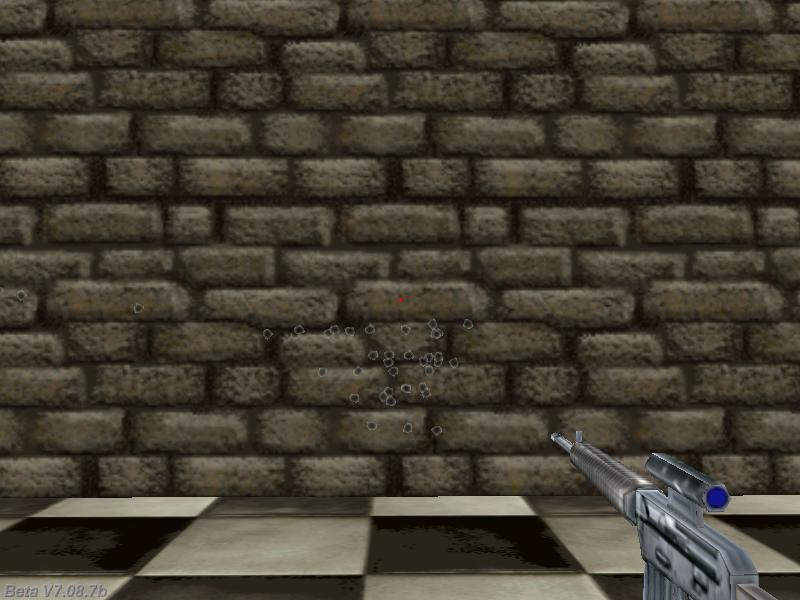Q: My script includes two gun entities. Setting their positions works fine, but I want to be able to change the gun model as well, depending on the value of a variable named currentGun. How can I do that? A: Here's an example that changes the guns when the "1" or "2" keys are pressed, or when currentGun is set to 1 or 2.   var currentGun = 1; // default weapon = no.1   ENTITY* weapon1_ent = {        type = "weapon1.mdl"; // weapon model        pan = 0; // weapon angle        x = 55; // 55 quants ahead of the view, play with this value        y = -20; // 20 quants towards the right side of the screen, play with this value        z = -20; // 20 quants below, play with this value        pan = 2; // weapon's pan angle (you can also use tilt and roll) }   ENTITY* weapon2_ent = {        type = "weapon2.mdl"; // weapon model        pan = 0; // weapon angle        x = 60; // 60 quants ahead of the view, play with this value        y = 15; // 15 quants towards the left side of the screen, play with this value        z = -18; // 18 quants below, play with this value        pan = -3; // weapon's pan angle (you can also use tilt and roll) }   function toggle_guns_startup() {        while (1)        {                if (key_1)                {                        weapon1_ent.flags2 = VISIBLE;                        weapon2_ent.flags2 = ~VISIBLE;                        currentGun = 1;                }                if (key_2)                {                        weapon1_ent.flags2 = ~VISIBLE;                        weapon2_ent.flags2 = VISIBLE;                        currentGun = 2;                }                if (currentGun == 1)                {                        weapon1_ent.flags2 = VISIBLE;                        weapon2_ent.flags2 = ~VISIBLE;                                        }                if (currentGun == 2)                {                        weapon1_ent.flags2 = ~VISIBLE;                        weapon2_ent.flags2 = VISIBLE;                }                wait (1);        } }     Q: I am making my own version of Guitar Hero. I have already made a simple level and some note models. The level and the songs work, but I don't want to make a new level for each and every song, on each and every difficulty, on each and every instrument. Is it possible to make a file (like a text file, a script file or whatever) that contains the positions of the notes and how many neckboard pieces are needed, which can be opened by a script that loads the information and displays the notes and neckboard on the screen and also assigns the notes and the neckboard their actions? A: Check out my dungeon creator from Aum54 - it does everything you need (and a bit more).     Q: I want to create a different starfield effect; I want to have tiny white pixels. Creating a skycube produces blurred pixel dots; I want to have sharp white points. The stars cannot move while I move my ship, since they are very very far. So I need them to be created and just blink a little, following the player but without any trail. A: Here's an example that fades in the stars, keeps them on for a while and then fades them out.   BMAP* particle_tga = "particle.tga";   function fade_particle(PARTICLE *p) {        p.alpha += 0.5 * time_step; // fade in the particles        if (p.alpha > 50) // they are fully visible?        {                p.alpha -= 1 * time_step; // then fade them out!        }        if (p.alpha < 0)                p.lifespan = 0; }   function particle_function(PARTICLE *p) {       p.alpha = 0;       p.bmap = particle_tga;       p.size = 5; // play with this value - it gives the size of the stars       p.event = fade_particle; }   action starfield() // attach this action to a high resolution sphere model {        // make sure that your player / ship action includes the line "player = my;"        while (!player) {wait (1);}        // set the scale for the starfield (the distance between player's ship and the stars)        vec_set(my.scale_x, vector(15, 15, 15));        set (my, PASSABLE | INVISIBLE);        var particle_pos;        while (1)        {                vec_set (my.x, player.x);                my.skill1 = 0;                // pick a random vertex to generate a new blinking star                my.skill1 = integer(random(ent_vertices (my))) + 1;             vec_for_vertex(particle_pos, my, my.skill1);             effect(particle_function, 1, particle_pos, nullvector);                  // add more lines like the one below if you want to have even more stars each frame                my.skill1 = integer(random(ent_vertices (my))) + 1;             vec_for_vertex(particle_pos, my, my.skill1);             effect(particle_function, 1, particle_pos, nullvector);                  my.skill1 = integer(random(ent_vertices (my))) + 1;             vec_for_vertex(particle_pos, my, my.skill1);             effect(particle_function, 1, particle_pos, nullvector);                  wait (1);        } }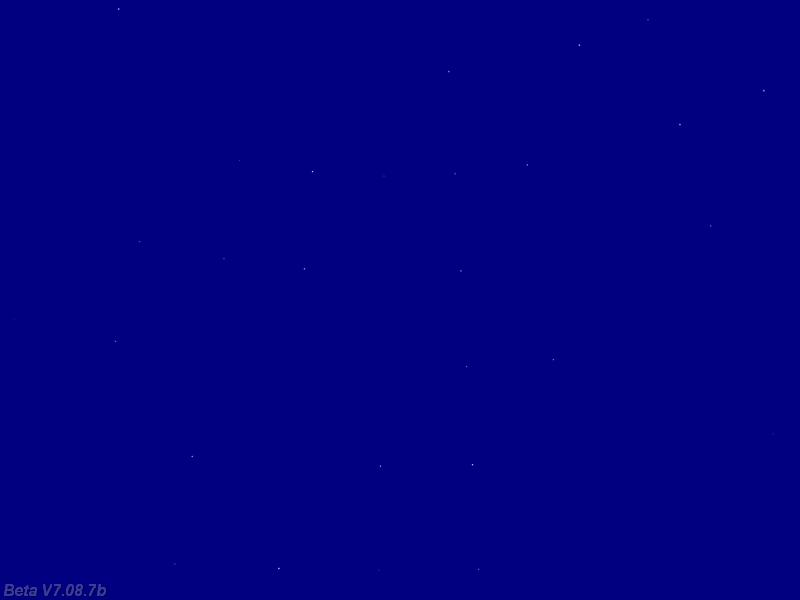Q: How do I make an object that when you walk up to it and press a key, it displays a message? A: Here you go.   TEXT* mymessage_txt = {        pos_x = 300;        pos_y = 50;        string("Hello there, stranger!"); }   action my_object() {        while (!player) {wait (1);}        while (1)        {                // if the player comes close to the object                if (vec_dist(player.x, my.x) < 100) // play with 100                {                        if (key_space)                        {                                while (key_space) {wait (1);} // wait until the space key is released                                set(mymessage_txt, VISIBLE); // and then display the message                                wait (-3); // for 3 seconds                                reset(mymessage_txt, VISIBLE); // now hide the message                        }                }                wait (1);        } }     Q: I have a 3D map and little dots to show the enemy ships' positions. I am using screen entities for the cube map and the dots; is there any way to assign a function to the dots? A: You can't attach an action to a screen entity, but you can control it using a function; here's an example:   ENTITY* dot1_ent = {        type = "dot.mdl";        x = 200;        y = 50;        z = 20;        flags2 = VISIBLE;                 }   ENTITY* dot2_ent = {        type = "dot.mdl";        x = 200;        y = 35;        z = 40;        flags2 = VISIBLE;                 }   ENTITY* dot3_ent = {        type = "dot.mdl";        x = 200;        y = 20;        z = 60;        flags2 = VISIBLE;                 }   // just an example that moves the dots randomly on the screen function dot1_startup() {        while (1)        {                dot1_ent.x += (1 - random(2)) * time_step;                dot1_ent.y += (1 - random(2)) * time_step;                wait (1);        }         }   function dot2_startup() {        while (1)        {                dot2_ent.x += (1 - random(2)) * time_step;                dot2_ent.y += (1 - random(2)) * time_step;                wait (1);        }         }   function dot3_startup() {        while (1)        {                dot3_ent.x += (1 - random(2)) * time_step;                dot3_ent.y += (1 - random(2)) * time_step;                wait (1);        }         }